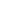# A sum of money at simple interest amounts to Rs.1568 in 4 years and to Rs. 1653.75 in 4 years. The sum is:

a) 1225
b) 1250
c) 950
d) 1500

 1225. Solution S.I. for 1 year = (1653.75 - 1568) = Rs. 85.75 S.I. for 4 years = Rs.(85.75 x 5) = Rs. 343 Principal = Rs. (1568 - 343) = Rs. 1225
Share :
+
Next
« Prev
Prev
Next »